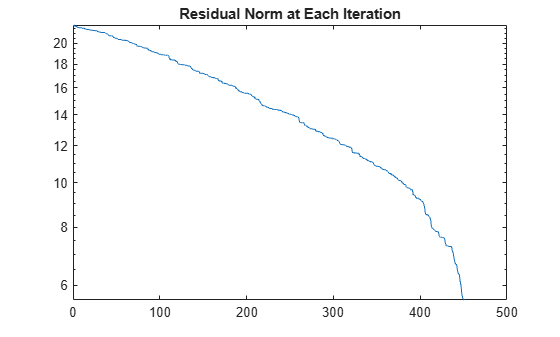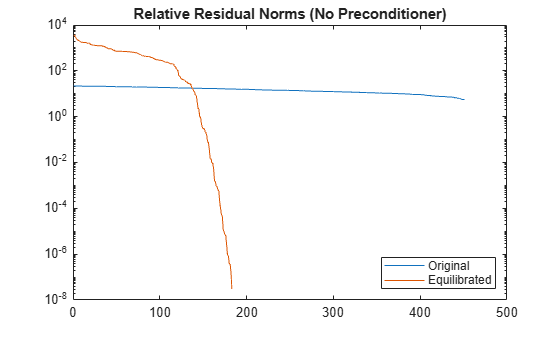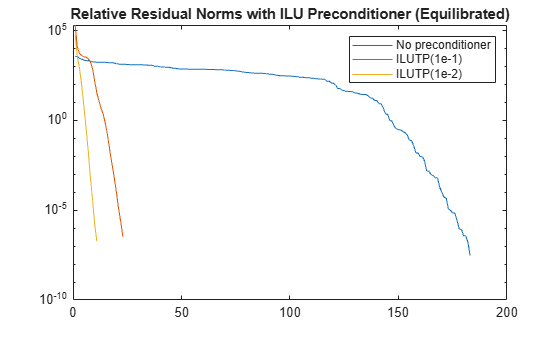# equilibrate

Matrix scaling for improved conditioning

Since R2019a

## Syntax

``[P,R,C] = equilibrate(A)``
``[P,R,C] = equilibrate(A,outputForm)``

## Description

example

````[P,R,C] = equilibrate(A)` permutes and rescales matrix `A` such that the new matrix ```B = R*P*A*C``` has a diagonal with entries of magnitude 1, and its off-diagonal entries are not greater than 1 in magnitude.```
````[P,R,C] = equilibrate(A,outputForm)` returns the outputs `P`, `R`, and `C` in the form specified by `outputForm`. For example, you can specify `outputForm` as `"vector"` to return the outputs as column vectors.```

## Examples

collapse all

Equilibrate a matrix with a large condition number to improve the efficiency and stability of a linear system solution with the iterative solver `gmres`.

Load the `west0479` matrix, which is a real-valued 479-by-479 sparse matrix. Use `condest` to calculate the estimated condition number of the matrix.

```load west0479 A = west0479; c1 = condest(A)```
```c1 = 1.4244e+12 ```

Try to solve the linear system $\mathrm{Ax}=\mathit{b}$ using `gmres` with 450 iterations and a tolerance of `1e-11`. Specify five outputs so that `gmres` returns the residual norms of the solution at each iteration (using `~` to suppress unneeded outputs). Plot the residual norms in a semilog plot. The plot shows that `gmres` is not able to achieve a reasonable residual norm, and so the calculated solution for $\mathit{x}$ is not reliable.

```b = ones(size(A,1),1); tol = 1e-11; maxit = 450; [x,flx,~,~,rvx] = gmres(A,b,[],tol,maxit); semilogy(rvx) title('Residual Norm at Each Iteration')```Use `equilibrate` to permute and rescale `A`. Create a new matrix `B = R*P*A*C`, which has a better condition number and diagonal entries of only 1 and -1.

```[P,R,C] = equilibrate(A); B = R*P*A*C; c2 = condest(B)```
```c2 = 5.1036e+04 ```

Using the outputs returned by `equilibrate`, you can reformulate the problem $\mathrm{Ax}=\mathit{b}$ into $\mathrm{By}=\mathit{d}$, where $\mathit{B}=\mathrm{RPAC}$ and $\mathit{d}=\mathrm{RPb}$. In this form you can recover the solution to the original system with $\mathit{x}=\mathrm{Cy}$. The recovered solution, however, might not have the desired residual error for the original system. See Rescaling to Solve a Linear System for details.

Use `gmres` to solve $\mathrm{By}=\mathit{d}$ for $\mathit{y}$, and then replot the residual norms at each iteration. The plot shows that equilibrating the matrix improves the stability of the problem, with `gmres` converging to the desired tolerance of `1e-11` in fewer than 200 iterations.

```d = R*P*b; [y,fly,~,~,rvy] = gmres(B,d,[],tol,maxit); hold on semilogy(rvy) legend('Original', 'Equilibrated', 'Location', 'southeast') title('Relative Residual Norms (No Preconditioner)') hold off```Improve Solution with Preconditioner

After you obtain the matrix `B`, you can improve the stability of the problem even further by calculating a preconditioner for use with `gmres`. The numerical properties of `B` are better than those of the original matrix `A`, so you should use the equilibrated matrix to calculate the preconditioner.

Calculate two different preconditioners with `ilu`, and use these as inputs to `gmres` to solve the problem again. Plot the residual norms at each iteration on the same plot as the equilibrated norms for comparison. The plot shows that calculating preconditioners with the equilibrated matrix greatly increases the stability of the problem, with `gmres` achieving the desired tolerance in fewer than 30 iterations.

```semilogy(rvy) hold on [L1,U1] = ilu(B,struct('type','ilutp','droptol',1e-1,'thresh',0)); [yp1,flyp1,~,~,rvyp1] = gmres(B,d,[],tol,maxit,L1,U1); semilogy(rvyp1) [L2,U2] = ilu(B,struct('type','ilutp','droptol',1e-2,'thresh',0)); [yp2,flyp2,~,~,rvyp2] = gmres(B,d,[],tol,maxit,L2,U2); semilogy(rvyp2) legend('No preconditioner', 'ILUTP(1e-1)', 'ILUTP(1e-2)') title('Relative Residual Norms with ILU Preconditioner (Equilibrated)') hold off```Create a 6-by-6 magic square matrix, and then use `equilibrate` to factor the matrix. By default, `equilibrate` returns the permutation and scaling factors as matrices.

`A = magic(6)`
```A = 6×6 35 1 6 26 19 24 3 32 7 21 23 25 31 9 2 22 27 20 8 28 33 17 10 15 30 5 34 12 14 16 4 36 29 13 18 11 ```
`[P,R,C] = equilibrate(A)`
```P = 6×6 0 0 0 0 1 0 0 0 0 0 0 1 0 0 0 1 0 0 1 0 0 0 0 0 0 0 1 0 0 0 0 1 0 0 0 0 ```
```R = 6×6 0.1852 0 0 0 0 0 0 0.1749 0 0 0 0 0 0 0.1909 0 0 0 0 0 0 0.1588 0 0 0 0 0 0 0.1793 0 0 0 0 0 0 0.1966 ```
```C = 6×6 0.1799 0 0 0 0 0 0 0.1588 0 0 0 0 0 0 0.1588 0 0 0 0 0 0 0.2422 0 0 0 0 0 0 0.2066 0 0 0 0 0 0 0.2035 ```

Construct the equilibrated matrix `Bmatrix` using the matrix factors `P`, `R`, and `C`.

`Bmatrix = R*P*A*C`
```Bmatrix = 6×6 1.0000 0.1471 1.0000 0.5385 0.5358 0.6031 0.1259 1.0000 0.8056 0.5509 0.6506 0.3916 0.2747 0.8485 1.0000 0.7859 0.3943 0.5825 1.0000 0.0252 0.1513 1.0000 0.6233 0.7754 1.0000 0.2562 0.0569 0.9553 1.0000 0.7295 0.1061 0.9988 0.2185 1.0000 0.9341 1.0000 ```

Now, specify the `"vector"` option to return the outputs as vectors. With large input matrices, returning the outputs as vectors can save memory and improve efficiency.

`[p,r,c] = equilibrate(A,"vector")`
```p = 6×1 5 6 4 1 3 2 ```
```r = 6×1 0.1852 0.1749 0.1909 0.1588 0.1793 0.1966 ```
```c = 6×1 0.1799 0.1588 0.1588 0.2422 0.2066 0.2035 ```

Construct the equilibrated matrix `Bvector` using the vector outputs `p`, `r`, and `c`.

`Bvector = r.*A(p,:).*c'`
```Bvector = 6×6 1.0000 0.1471 1.0000 0.5385 0.5358 0.6031 0.1259 1.0000 0.8056 0.5509 0.6506 0.3916 0.2747 0.8485 1.0000 0.7859 0.3943 0.5825 1.0000 0.0252 0.1513 1.0000 0.6233 0.7754 1.0000 0.2562 0.0569 0.9553 1.0000 0.7295 0.1061 0.9988 0.2185 1.0000 0.9341 1.0000 ```

Compare `Bvector` and `Bmatrix`. The result indicates they are equal.

`norm(Bmatrix - Bvector)`
```ans = 0 ```

## Input Arguments

collapse all

Input matrix, specified as a square matrix. `A` can be dense or sparse, but must be structurally nonsingular, as determined by `sprank`.

When `A` is sparse, the outputs `P`, `R`, and `C` are also sparse.

Data Types: `single` | `double`
Complex Number Support: Yes

Output format, specified as `"vector"` or `"matrix"`. This option allows you to specify whether the outputs `P`, `R`, and `C` are returned as column vectors or as matrices:

• `"matrix"``P`, `R`, and `C` are matrices such that ```B = R*P*A*C```.

• `"vector"``P`, `R`, and `C` are column vectors such that ```B = R.*A(P,:).*C'```.

Example: `[P,R,C] = equilibrate(A,"vector")` returns `P`, `R`, and `C` as vectors.

## Output Arguments

collapse all

Permutation information, returned as a matrix or vector. `P*A` (or `A(P,:)` with the `"vector"` option) is the permutation of `A` that maximizes the absolute value of the product of its diagonal elements.

Row scaling, returned as a diagonal matrix or vector. The nonzero entries in `R` and `C` are real and positive.

Column scaling, returned as a diagonal matrix or vector. The nonzero entries in `R` and `C` are real and positive.

For linear system solutions `x = A\b`, the condition number of `A` is important for accuracy and efficiency of the calculation. `equilibrate` can improve the condition number of `A` by rescaling the basis vectors. This effectively forms a new coordinate system that both `b` and `x` can be expressed in.
`equilibrate` is most useful when the scales of the `b` and `x` vectors in the original system ```x = A\b``` are irrelevant. However, if the scales of `b` and `x` are relevant, then using `equilibrate` to rescale `A` only for the linear system solve is not recommended. The obtained solution does not generally yield a small residual for the original system, even if expressed using the original basis vectors.